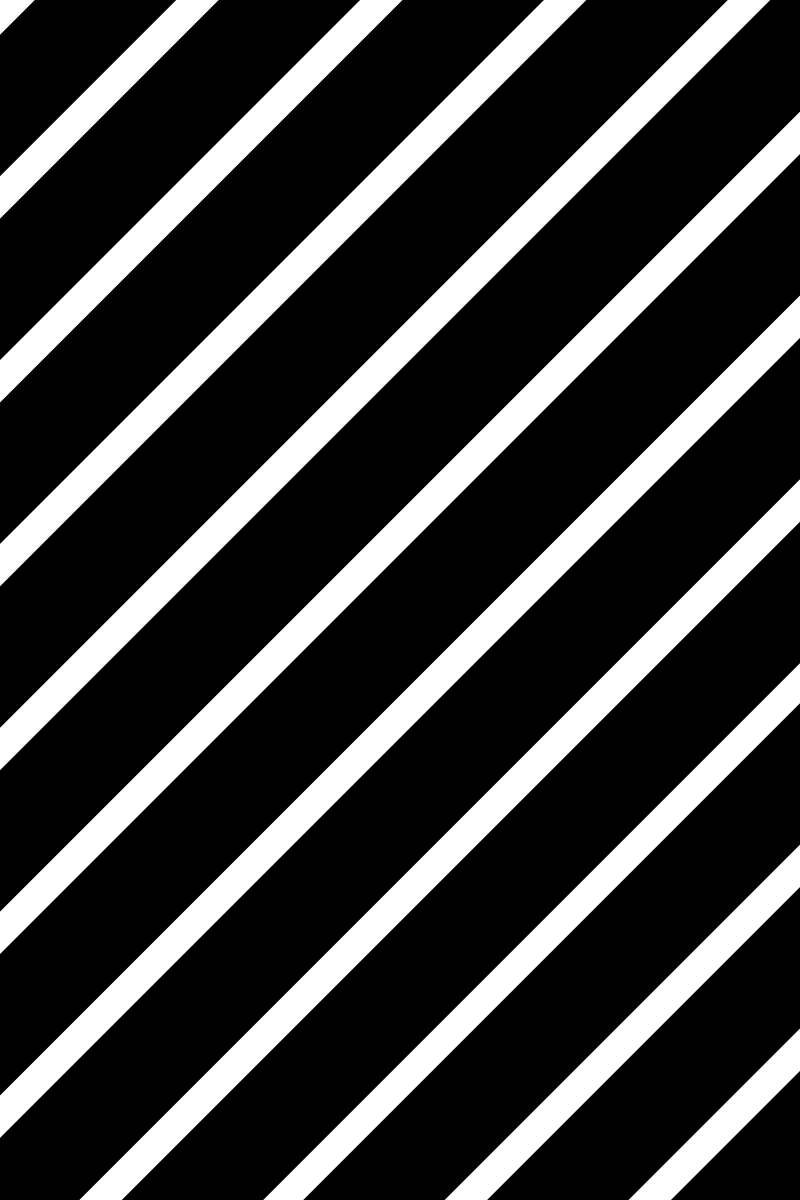We covered the use of the clip-path property for clipping using CSS, so it’s only natural that we now go over masking. Contrary to clipping, where a part of an image or element is either completely invisible or completely visible, with masking we can hide or show parts of an image with different levels of opacity.

Let’s first use a simple linear gradient that goes from transparent to black. The first image is our default starting image, and the second image has our linear gradient applied as the mask-image value:Here’s the CSS rules used here:

``````.mask1 {
}``````

Here are two more examples of interesting effects that can be accomplished with masking using gradients:``````.mask2 {
}
}``````

Here’s we’re using an image that was created using Sketch as our image mask. The first image is the image mask itself, and the second image has that mask applied to it:And our CSS looks like this:

``````.mask4 {
}``````

We specified a value for mask-size here because our image mask is 800px by 1200px, but here we want everything shrunk by half so that the image can look sharp on retina displays.

The first example currently only seems to be working in Firefox (you probably won’t see anything in non-supporting browsers). It defines the SVG mask and then we reference the ID of the mask in CSS as usual. The second example seems to have wider support and defines the image as part of the SVG element itself.

Also note that with SVG masks, the colors to use are white and black instead of transparent and black. The colors also work in reverse and white/partially white is what will be visible.### Example 1 (triangle)

Here’s the SVG markup for the first example:

``````<svg width="0" height="0" viewBox="0 0 400 600">
<defs>
<rect fill="#000000" x="0" y="0" width="400" height="600"></rect>
<polygon fill="#FFFFFF" points="200.5 152 349 449 52 449"></polygon>
</defs>
</svg>
``````

Then we can apply the mask to our image with mask-image as usual by refecencing the ID of the SVG mask:

``````.mask5 {
}``````

### Example 2 (bubbles)

For our second SVG example, everything is contained in the SVG definition, including our main image itself:

``````<svg width="400px" height="600px" viewBox="0 0 400 600">
<defs>
<rect id="Rectangle" fill="#000000" x="0" y="0" width="400" height="600"></rect>
<circle id="Oval" fill="#FFFFFF" cx="67.5" cy="51.5" r="67.5"></circle>
<circle id="Oval" fill="#FFFFFF" cx="296.597656" cy="118.597656" r="56.5976562"></circle>
<circle id="Oval" fill="#FFFFFF" cx="53.4648437" cy="256.464844" r="81.4648437"></circle>
<circle id="Oval" fill="#FFFFFF" cx="239.587891" cy="313.587891" r="70.5878906"></circle>
<circle id="Oval" fill="#FFFFFF" cx="366.597656" cy="562.597656" r="56.5976562"></circle>
<circle id="Oval" fill="#FFFFFF" cx="93.203125" cy="486.203125" r="76.203125"></circle>